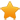Switch Editions?
CancelChannel: CodeSection,代码区,网络安全 - CodeSec
Viewing all articles

# 基于Pari数学软件浅谈RSA算法相关密码题型（基础篇）

0
0

0×00 绪论

0×01 RSA算法简介

RSA算法是1978年由Rivest, Shamir和 Adlemen这三位密码学大牛基于大数分解这一困难问题而设计的公钥密码算法，先后被ISO、ITU、 SWIFT等国际化标准组织采纳为国际标准，被广泛应用于加密密钥、数字签名、身份认证、协议设计等领域。

RSA算法又称为RSA公开密钥密码体制――所谓的公开密钥密码体制就是使用不同的加密 密钥 与解密密钥，是一种“由已知加密密钥推导出解密密钥在计算上是不可行的” 密码体制。RSA是被研究得最广泛的 公钥 算法，从提出到现在的三十多年里，经历了各种攻击的考验，逐渐为人们接受，普遍认为是目前最优秀的公钥方案之一。

RSA算法是一种非对称密码算法，所谓非对称，就是指该算法需要一对 密钥 ，使用其中一个加密，则需要用另一个才能解密。

RSA算法涉及的参数有n、p、q 、e1、e2等。

RSA加解密的算法完全相同，设A为明文，B为密文，则：

A=B^e1 mod n；B=A^e2 mod n；

e1和e2可以互换使用，即：

A=B^e2 mod n；B=A^e1 mod n; 0×02 Pari 简介

0×03举个栗子

e1=463884209889475377478742046340962125043690799298268667047773，

B=825568930611462903672838325065041844731508649912306161613952,

1) 给出N的分解；

2) 求私钥；

3) 求B对应的明文

A=B^e2 mod N；B=A^e1 mod N

Quick_Mod(x, n, N) =
{
bin_n= binary(n);
len= length(bin_n);
result= 1;
power_x= Mod(x, N);
for(i= 1, len,
if(bin_n[len+ 1 - i],
result*= power_x;
);
power_x= power_x^2;
);
return(lift(lift(result)));
}

Quick_Mod(x, n, N)= { bin_n =binary(n); len = length(bin_n); result = 1; power_x = Mod(x, N); for(i = 1,len,if(bin_n[len + 1 - i],result *= power_x; ); power_x = power_x^2; );return(lift(lift(result))); }

Viewing all articles

More Pages to Explore .....